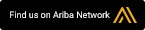# Articles

## Equivalence Partitioning

To celebrate completion of the update to Advanced Software Testing: Volume I, here is an excerpt of Chapter 3. This is the central chapter of the book, addressing test design techniques.

We start with the most basic of specification-based test design techniques, equivalence partitioning.

Conceptually, equivalence partitioning is about testing various groups that are expected to be handled the same way by the system and exhibit similar behavior.  Those groups can be inputs, outputs, internal values, calculations, or time values, and should include valid and invalid groups. We select a single value from each equivalence partition, and this allows us to reduce the number of tests.  We can calculate coverage by dividing the number of equivalence partitions tested by the number identified, though generally the goal is to achieve 100% coverage by selecting at least one value from each partition.

This technique is universally applicable at any test level, in any situation where we can identify the equivalence partitions. Ideally, those partitions are independent, though some amount of interaction between input values does not preclude the use of the technique. This technique is also very useful in constructing smoke tests, though testing of some of the less-risky partitions frequently is omitted in smoke tests. This technique will find primarily functional defects where data is processed improperly in one or more partitions. The key to this technique is to take care that the values in each equivalence partition are indeed handled the same way; otherwise, you will miss potentially important test values.